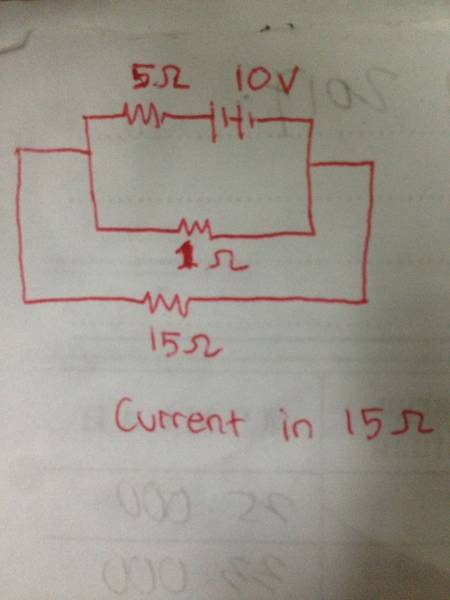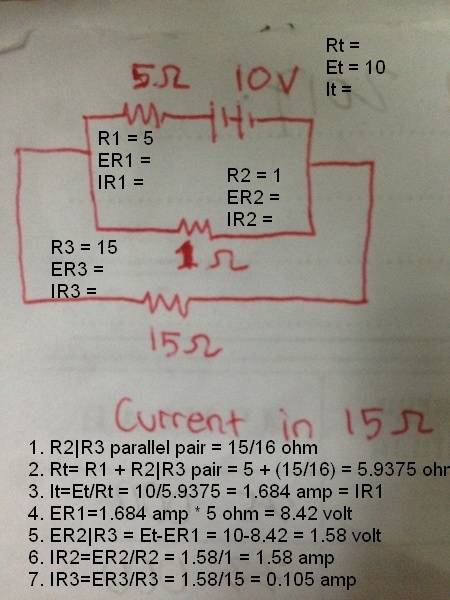# Can you help me find the current through the 15 Ohm resistor branch?

Helly123

## Homework Statement## Homework Equations

I = VR
Series = current the same, voltage different
Parallel = current different, voltage the same

## The Attempt at a Solution

Is the 1 ohm and 15 ohm parallel? I'm still learning to differentiate it.
If yes, then R = 15/16 ohm
Is the R series to 5ohm? If yes, R total become 95/16 ohm
I = 10 / (95/16) = 160/95 A = 1,68A

For Current in resistor 15 = 15/16 x 1,68 A = 1,58 A

Is it correct?

#### Attachments

Last edited:

Asymptotic
Is the 1 ohm and 15 ohm parallel? I'm still learning to differentiate it.
If yes, then R = 15/16 ohm
Yes.
For Current in resistor 15 = 15/16 x 1,68 A = 1,58 A

Is it correct?
No.

Helly123
No.

V for 15and1ohm = I.R = 1.68 x 15/16 = 1.57 v
I for 15 = V/R = 1.57 v / 15 ohm = 0.105 A

Asymptotic
V for 15and1ohm = I.R = 1.68 x 15/16 = 1.57 v
I for 15 = V/R = 1.57 v / 15 ohm = 0.105 A
Yes.
Do you understand, and can you explain why this answer is correct, and the previous answer was wrong?

•Helly123
Helly123
Yes.
Do you understand, and can you explain why this answer is correct, and the previous answer was wrong?
maybe because, the current cannot be calculated according to the fraction of total resistance.

Homework Helper
Gold Member
2022 Award
maybe because, the current cannot be calculated according to the fraction of total resistance.
It can, but not quite as you did it. Would you expect the larger resistance to have the greater current or the lesser?

•Asymptotic and Helly123
Helly123
It can, but not quite as you did it. Would you expect the larger resistance to have the greater current or the lesser?
According to formula I = V/R
The greater the resistance the lesser the current.. to be honest, i try to think why the first one is wrong...
the total R for 15 and 1 ohm are 15/16 ohm
And the use the fraction method R1/Rtotal * I total
15/(15/16) * 1.68 A
But its wrong.. why is that..?

Asymptotic
Since you've gotten the correct answers, but are not quite sure from whence they've come, allow me to suggest a methodology I call the "fully canonical with integrated smell test" approach.

Step 1: Label everything on the drawing including all the known values.
Step 2: Now begins an iterative procedure of identifying subsets of the overall problem that can be solved, solving them, updating the drawing with this new information, and leveraging each increase in circuit knowledge to solve for the other unknowns.
Step 3: Smell test phase. After all the voltages and currents have been solved for, and the drawing has been marked up with these values, inspect the drawing for obvious inconsistencies. In this example, R2 and R3 are a parallel pair, and by definition R2 and R3 must have the same voltage dropped across them - if they don't, something has gone wrong. In a similar vein, the current through R1 must be the same as total current, voltage across R1 added to voltage across the R2|R3 parallel pair must add up to total voltage, and so on.Doing it this way is a fair amount of work, and isn't as valuable a technique after one gets the hang of how to solve circuits, but is a useful 'sanity check' for beginners.

#### Attachments

•Helly123
Homework Helper
Gold Member
2022 Award
According to formula I = V/R
The greater the resistance the lesser the current.. to be honest, i try to think why the first one is wrong...
the total R for 15 and 1 ohm are 15/16 ohm
And the use the fraction method R1/Rtotal * I total
15/(15/16) * 1.68 A
But its wrong.. why is that..?
I=V(1/R1+1/R2)=V/Rnet
I1=V/R1
I1/I=?/Rnet
Deduce what ? Is.

Helly123
Since you've gotten the correct answers, but are not quite sure from whence they've come, allow me to suggest a methodology I call the "fully canonical with integrated smell test" approach.

Step 1: Label everything on the drawing including all the known values.
Step 2: Now begins an iterative procedure of identifying subsets of the overall problem that can be solved, solving them, updating the drawing with this new information, and leveraging each increase in circuit knowledge to solve for the other unknowns.
Step 3: Smell test phase. After all the voltages and currents have been solved for, and the drawing has been marked up with these values, inspect the drawing for obvious inconsistencies. In this example, R2 and R3 are a parallel pair, and by definition R2 and R3 must have the same voltage dropped across them - if they don't, something has gone wrong. In a similar vein, the current through R1 must be the same as total current, voltage across R1 added to voltage across the R2|R3 parallel pair must add up to total voltage, and so on.

View attachment 216833

Doing it this way is a fair amount of work, and isn't as valuable a technique after one gets the hang of how to solve circuits, but is a useful 'sanity check' for beginners.
I get it! 15 and 1 are parallel. Fractions used in series circuit , since total R = R1+R2...etc
So, in 15 ohm wire, resistance series to it is only 15 ohm itself, so the fraction is 15/15 instead of 15/(15/16)

Helly123
I=V(1/R1+1/R2)=V/Rnet
I1=V/R1
I1/I=?/Rnet
Deduce what ? Is.
Maybe I1 = (Rnet/R1) * I net
Since I and R is inversely propotional

Last edited:
Asymptotic
I'm not quite following.
15 and 1 are parallel.
Yes, they are.
Fractions used in series circuit , since total R = R1+R2...etc
I don't get this. "R = R1+R2...etc" is true for a series circuit, but the values aren't necessarily fractions.
So, in 15 ohm wire, resistance series to it is only 15 ohm itself, so the fraction is 15/15 instead of 15/(15/16)
I'm not following this at all.

Helly123
I'm not quite following.

Yes, they are.

I don't get this. "R = R1+R2...etc" is true for a series circuit, but the values aren't necessarily fractions.
I'm not following this at all.
I missed something.. i meant
I = V * R
Whereas the R is 15ohm

V is the voltage of 1&15 ohm parallel pair.

Homework Helper
Gold Member
2022 Award
Maybe I1 = (Rnet/R1) * I net
Since I and R is inversely propotional
Right, since V=I1R1=I2R2=InetRnet.
This is the inverse of what you had in post #7.

•Asymptotic and Helly123
Helly123
Right, since V=I1R1=I2R2=InetRnet.
This is the inverse of what you had in post #7.
Yes, i missed that before. Thanks